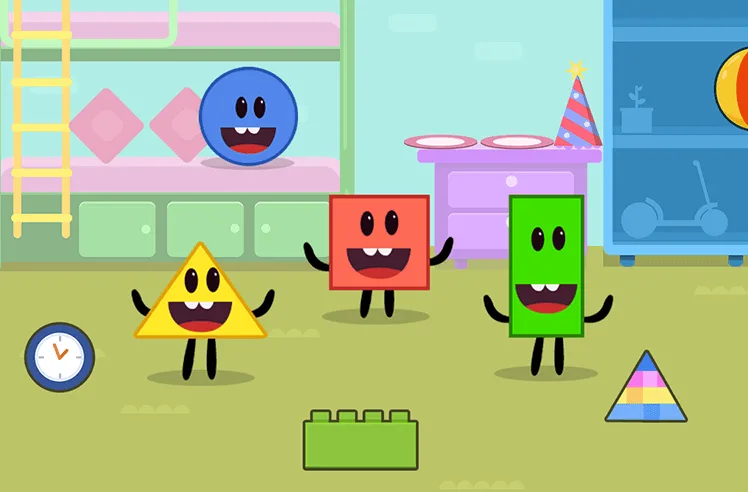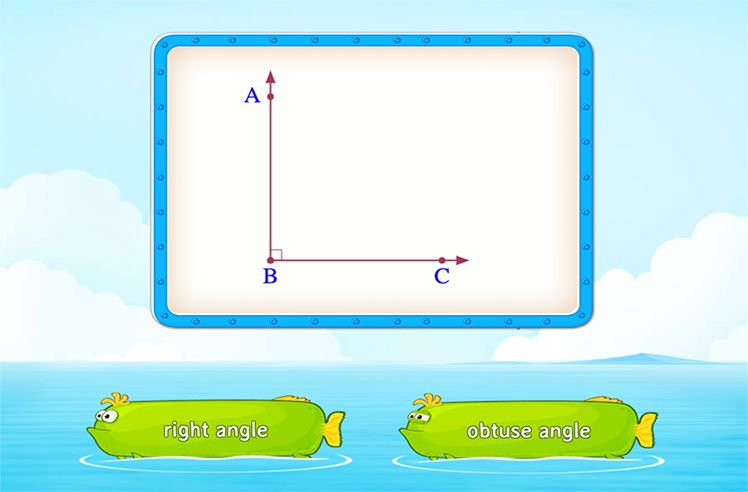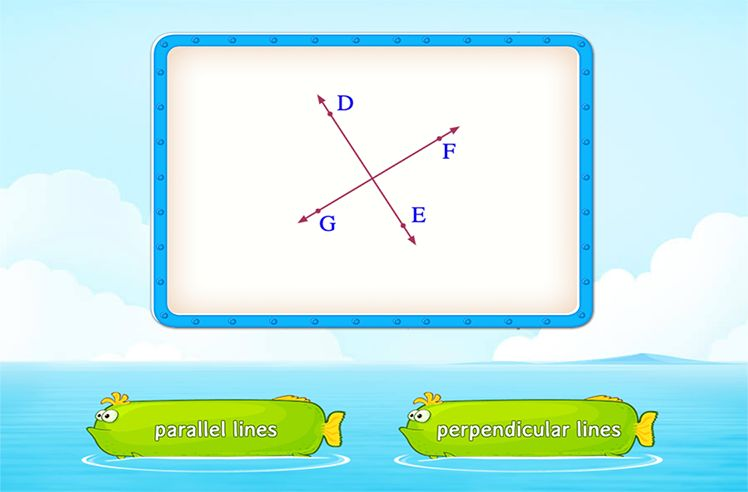# Picture Graph – Definition with Examples

» Picture Graph – Definition with Examples

## What is Picture Graph?

A picture graph uses symbols and pictures to represent data.

The pictograph shows data on the number of pens sold on each day over a week by a store.

The key shows that 1 picture of a pen shows 4 pens were sold, which means that the scale of the pictograph is 4. A scale implies the number of objects shown by 1 symbol or picture on the graph.

The picture of half a pen means 2 pens were sold.

On the above pictograph, there are 4 pens shown on Monday. As each pen represents 4 pens, that means, on Monday 4 × 4 = 16 pens were sold.

Similarly, the number of pens sold:

on Tuesday 5 × 4 = 20 pens

on Wednesday 3 × 4 = 12 pens

on Thursday 5 × 4 = 20 pens

on Friday, it shows a picture of 2 and a half pen. So, 2 pens represent 8 pens and half a pen represents 2 pens. Therefore,pens sold on Friday 8 + 2 = 10 pens.

On Saturday 6 × 4 = 24 pens

On Sunday 3 × 4 = 12 pens

## Drawing a Picture Graph

We can also draw a picture graph for a given set of data.

Here, is the data showing the number of children who visited a library during the first three months of a year.

To represent this data on a pictograph, follow the given steps:

• Think of a picture or a symbol to represent the children.
• Then, decide on a suitable scale to represent the number of children. For a scale of 10, each symbol or picture would represent 10 children.

Mention the key (the scale used).

So, taking a scale of 10, we get the picture graph as:

Some limitations of a picture graph:

Parts of a symbol used does not give exact data in case of large numbers. It can only be used for data given in whole numbers majorly. It is not very useful for larger data that numbers in hundreds or thousands or more.Shapes

Play NowAngles

Play NowParallel and Perpendicular Lines

Play Now